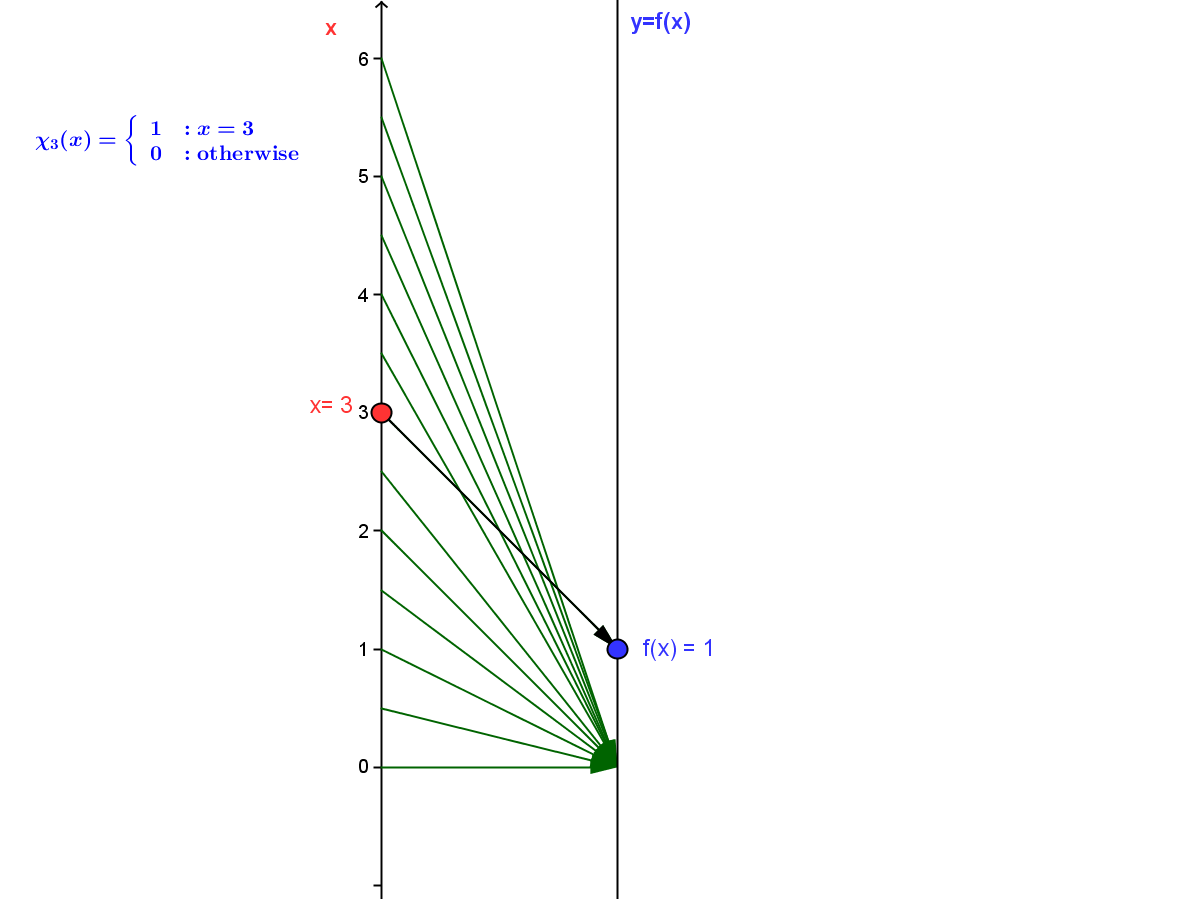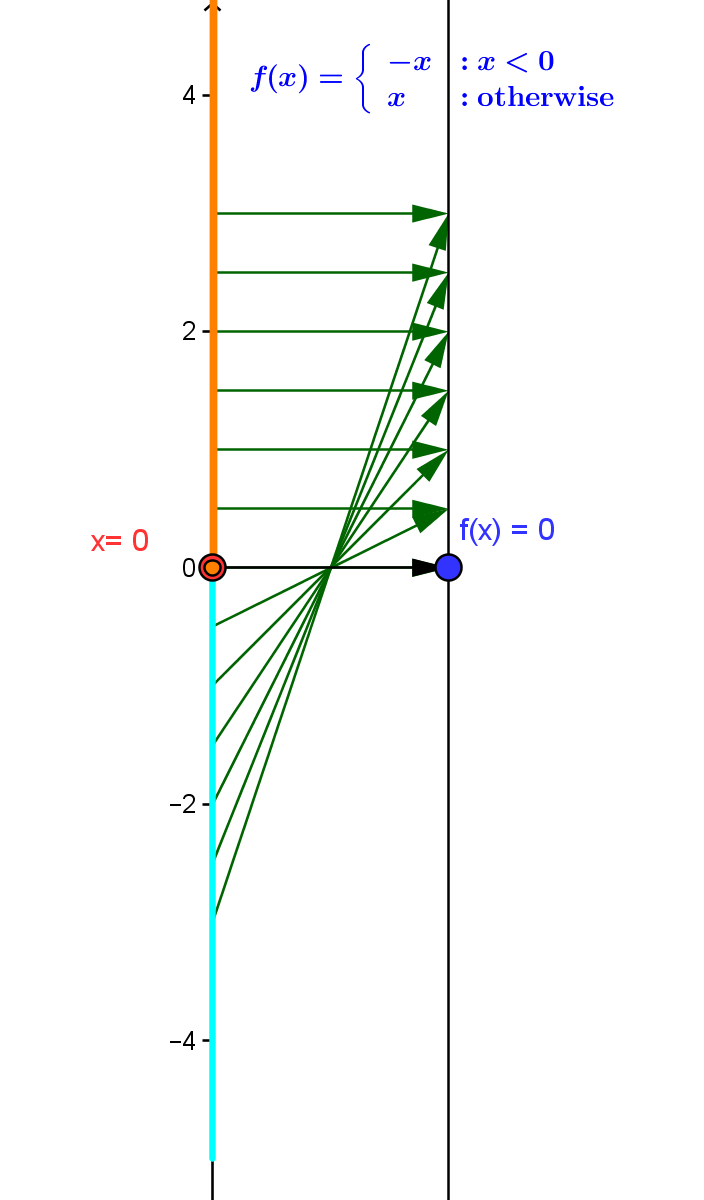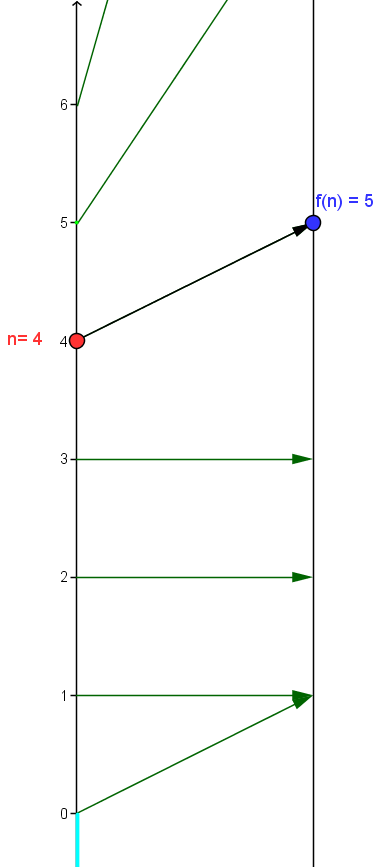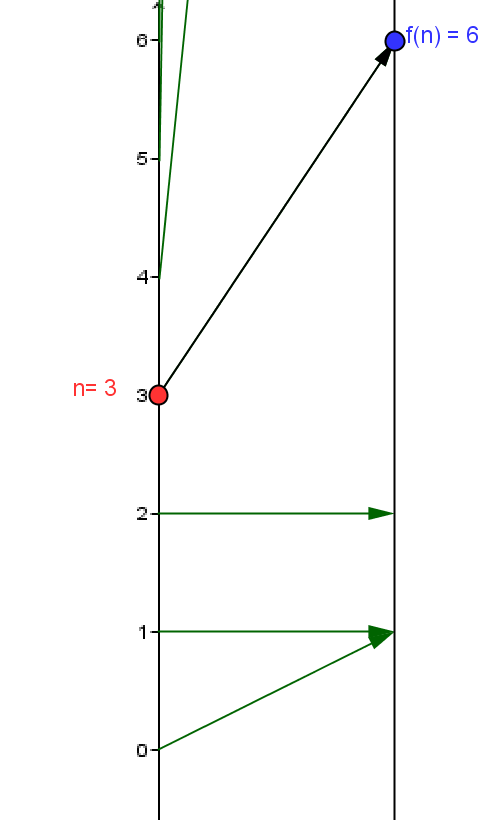### SectionOWOther Algebraic Functions

Multi-variable Functions and Calculus

Introduction: The concept of a function can be applied to have more than two variables interacting. One common application of the function concept has two, three or "$n$" variables controlling a single variable: $f: R^n \to R$.

Another application has a single variable controlling two, three or "$k$" variables.: $f: R \to R^k$.

Even more complicated is a function that uses two, three, or "$n$" variables to control two, three, or "$k$" other variables: $f: R^n \to R^k$.

The methods for studying multi-variable functions can become quite abstract when a total of more than three variables are involved. In part this is due to the limitations of visualizing more than three variables within visual constraints three dimensional cartesian coordinates.

This limitation for graphical visualization basically restricts the study of multi-variable functions to $f: R^n \to R^k$ where $n = 2$ and $k = 1$ or $n=1$ and $k = 2$.

A multi-variable function is defined by techniques similar to those used to define single real valued functions of a single variable.  The tools for visualizing these functions beyond the constraints of three dimensional cartesian geometry to investigate the wide range of related  concepts of continuity, differentiability, and integrability can benefit much from the use of mapping diagrams.

The key idea in visualizing multi-variable functions with mapping diagrams is to have $n+k$ parallel number lines (or axes),"n" lines  representing the source (input, controlling or independent) variable values and the other $k$ lines representing the target (output, controlled or dependent) variable values. The function is visualized by arrows that relate points (numbers) on these parallel axes.

#### Mapping Diagram Definition

Using some dynamic technology with GeoGebra we can see connections between the graph and mapping diagram visualizations.

#### Connecting Mapping Diagrams and Graphs

Example TMD.0 The First Example.
This example presents a single function using an algebraic formula, a table of data,  a graph and a mapping diagram.
You can download and try the worksheet for this section now: Worksheet.VF1.pdf.

#### OW.FDPC Function Definition by Piecewise Cases

Functions defined by piecewise cases are characterized by partitioning the domain of the function and defining the function value by identifying in what particular subset of the partition a number lies. Wikipedia on piecewise-defined functions.

A very simple example of a function defined by piecewise cases is the function $\chi_3$ where $\chi_3(3) = 1$ and $\chi_3(x) = 0$ for any $x \ne 3$.
[This function $\chi _3$  is described as "the characteristic function of the number $3$."]Mapping Diagram for $\chi_3$

Another important example of a function defined by piecewise cases is the function $f(x)= |x|$ where $f(x) = x$ when $x \ge 0$ and $f(x)=-x$ when $x < 0$.Mapping Diagram for $f(x) = |x|$
Functions defined by piecewise cases are abundant and important in many applications.

OW.FDINV Function Definition by Inversion

We have already discussed inverse functions and mapping diagrams in the section LF.INV on inverses of linear functions. See also Wikipedia on Inverse Functions.

We investigate definition by inversion further by exploring the core positive power functions $P_n(x) = x^n$ and their "inverses. "

Comment: Consider the core power function $P_3$ where $P_3(x) =x^3$.  This  function has a unique inverse function which we denote by $InvP_3$ or $P_3 ^{-1}$. It is commonly know as the "cube root" function so $InvP_3(x)= P_3 ^{-1}(x) = \sqrt x$ . The property that defines $invP_3=P_3 ^{-1}$ is  that $P_3(P_3 ^{-1}(x)) = (\sqrt x)^3 = x$ for all numbers $x$. We can also understand this by saying $P_3 ^{-1}(s)$ is defined as the unique number $t$ where $P_3(t) = t^3 =s$.

Applications of this method are studied extensively in intermediate algebra (for logarithms) and trigonometry ( for inverse trig functions).

#### OW.FDImpl  Implicit Function Definition

Functions implicitly defined by an equation are not mentioned in beginning algebra courses but occur quietly in the background connections between linear (line) and quadratic (conic curve) equations and functions. Wikipedia on Implicit Functions.

1. A very revealing example is given by recognizing an inverse function for the function $f$ is an implicit function for the equation $f(y)-x = 0$.
2. A commonly encountered example is given by recognizing that  the function $f(x) = \sqrt{ 1- x^2}$ for $x \in [-1,1]$  is an implicit function for the equation $x^2 +y^2 - 1 = 0$.

Example OW.0  An Implicit (Piecewise) Case Defined Function.

This example presents a (piecewise) case defined function $f$, implicitly defined with respect to the equation $x^2 +y^2 -4 = 0$ with a table of data,  a graph and a mapping diagram.

#### OW.FDRec  Recursive Function Definition

Functions defined recursively  (inductively) on subsets of the integers or related subsets of the real numbers are usually not mentioned directly in algebra courses, yet are encountered through experience in these and later courses both with sequences of numbers and of functions. Wikipedia on Recursive Definition of Functions

Examples of recursive functions $f: N \rightarrow R$ include the factorial function defined by $f(0)= 1$ and $f(n+1) = (n+1) \times f(n)$ for all $n > 0$ and  the Fibonacci sequence function, defined by $f(0)=0$, $f(1)= 1$ and $f(n+2) = f(n) +f(n+1)$. Both these functions can be visualized by mapping diagrams.

Fibonacci Function
 $n$ $f(n+2) = f(n) +f(n+1)$ 0 1 1 1 2 2 3 3 4 5 5 8Factorial Function
 $n$ $f(n+1)=(n+1)*f(n)$ 0 1 1 1 2 2 3 6 4 24 5 120Treatment of functions defined by cases and by inverses with their graphical interpretation is common in algebra courses in preparation for calculus.
Functions defined implicitly by equations or recursively are not mentioned much, if at all, before a first course in calculus.

What is missing is a balanced treatment using mapping diagrams to reinforce the function aspect in the visualizations of these other ways to define functions.  That will be emphasis of this section. It will provide examples that will help students use the power of the mapping diagram along with the three other tools (equations, tables , and graphs) to understand these other ways to define functions.